Tamil Nadu Board of Secondary EducationHSC Science Class 12th

# Tamil Nadu Board Samacheer Kalvi solutions for Class 12th Physics Volume 1 and 2 Answers Guide chapter 3 - Magnetism and magnetic effects of electric current [Latest edition]

#### Chapters## Chapter 3: Magnetism and magnetic effects of electric current

Evaluation
Evaluation [Pages 189 - 193]

### Tamil Nadu Board Samacheer Kalvi solutions for Class 12th Physics Volume 1 and 2 Answers Guide Chapter 3 Magnetism and magnetic effects of electric current Evaluation [Pages 189 - 193]

#### Multiple choice questions

Evaluation | Q I. 1. | Page 189

The magnetic field at the centre O of the following current loop is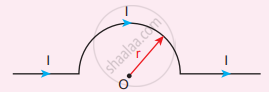• (mu_circ "I")/(4"r")⊗

• (mu_circ "I")/(4"r")⊙

• (mu_circ "I")/(2"r")⊗

• (mu_circ "I")/(2"r")⊙

Evaluation | Q I. 2. | Page 189

An electron moves straight inside a charged parallel plate capacitor of uniform charge density σ The time taken by the electron to cross the parallel plate capacitor when the plates of the capacitor are kept under constant magnetic field of induction vec"B" is-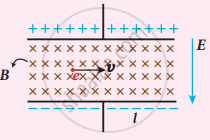• epsilon_circ "elB"/sigma

• epsilon_circ "lB"/(sigmal)

• epsilon_circ "lB"/("e"sigma)

• epsilon_circ "lB"/sigma

Evaluation | Q I. 3. | Page 189

A particle having mass m and charge q accelerated through a potential difference V. Find the force experienced when it is kept under perpendicular magnetic field B is

• sqrt((2"q"^3"BV")/"m")

• sqrt(("q"^3"B"^2"V")/"2m")

• sqrt(("2q"^3"B"^2"V")/"m")

• sqrt(("2q"^3"B""V")/"m"^3)

Evaluation | Q I. 4. | Page 188

A circular coil of radius 5 cm and 50 turns carries a current of 3 ampere. The magnetic dipole moment of the coil is nearly

• 1.0 Am2

• 1.2 Am2

• 0.5 Am2

• 0.8 Am2

Evaluation | Q I. 5. | Page 189

A thin insulated wire forms a plane spiral of N = 100 tight turns carrying a current 1 = 8 m A (milli ampere). The radii of inside and outside turns are a = 50 mm and b = 100 mm respectively. The magnetic induction at the center of the spiral is

• 5 μT

• 7 μT

• 8 μT

• 10 μT

Evaluation | Q I. 6. | Page 189

Three wires of equal lengths are bent in the form of loops. One of the loops is circle, another is a semi-circle and the third one is a square. They are placed in a uniform magnetic field and same electric current is passed through them. Which of the following loop configuration will experience greater torque?

• Circle

• Semi-circle

• Square

• All of them

Evaluation | Q I. 7. | Page 189

Two identical coils, each with N turns and radius R are placed coaxially at a distance R as shown in the figure. If I is the current passing through the loops in the same direction, then the magnetic field at a point P which is at exactly at "R"/2 distance between two coils is-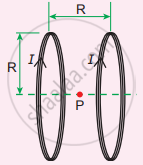• (8"N"mu_circ"I")/(sqrt5"R")

• (8"N"mu_circ"I")/(5^(3/2)"R")

• (8"N"mu_circ"I")/(5"R")

• (4"N"mu_circ"I")/(sqrt5"R")

Evaluation | Q I. 8. | Page 190

A wire of length l carrying a current I along the Y direction is kept in a magnetic field given by vec"B" = beta/sqrt3 (hat"i" + hat"j" + hat"k")T. The magnitude of Lorentz force acting on the wire is

• sqrt(2/3)beta"I"l

• sqrt(1/3)beta"I"l

• sqrt2 beta"I"l

• sqrt(1/2)beta"I"l

Evaluation | Q I. 9. | Page 190

A bar magnet of length l and magnetic moment pm is bent in the form of an arc as shown in figure. The new magnetic dipole moment will be-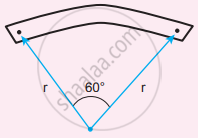• pm

• $\frac{3}{\pi}p_m$

• $\frac{2}{\pi}p_m$

• $\frac{1}{2}p_m$

Evaluation | Q I. 10. | Page 190

A non-conducting charged ring of charge q, mass m and radius r is rotated with constant angular speed ω. Find the ratio of its magnetic moment with angular momentum is

• "q"/"m"

• "2q"/"m"

• "q"/"2m"

• "q"/"4m"

Evaluation | Q I. 11. | Page 190

The BH curve for a ferromagnetic material is shown in the figure. The material is placed inside a long solenoid which contains 1000 turns/cm. The current that should be passed in the solenonid to demagnetize the ferromagnet completely is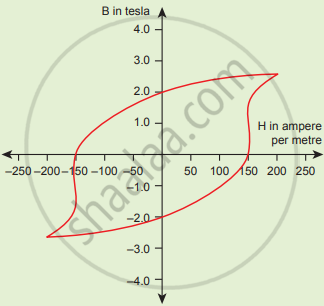• 1.00 m A

• 1.25 mA

• 1.50 mA

• 1.75 mA

Evaluation | Q I. 12. | Page 191

Two short bar magnets have magnetic moments 1.20 Am2 and 1.00 Am2respectively. They are kept on a horizontal table parallel to each other with their north poles pointing towards the south. They have a common magnetic equator and are separated by a distance of 20.0 cm. The value of the resultant horizontal magnetic induction at the mid-point O of the line joining their centers is (Horizontal components of Earth’s magnetic induction is 3.6 x 10-5 Wb m-2) (NSEP 2000-2001)

• 3.60 x 10-5 Wb m-1

• 3.5 x 10-5 Wb m-1

• 2.56 x 10-4 Wb m-1

• 2.2 x 10-4 Wb m-1

Evaluation | Q I. 13. | Page 191

The vertical component of Earth’s magnetic field at a place is equal to the horizontal component. What is the value of angle of dip at this place?

• 30°

• 45°

• 60°

• 90°

Evaluation | Q I. 14. | Page 191

A flat dielectric disc of radius R carries an excess charge on its surface. The surface charge density is σ. The disc rotates about an axis perpendicular to its plane passing through the centre with angular velocity ω. Find the magnitude of the torque on the disc if it is placed in a uniform magnetic field whose strength is B which is directed perpendicular to the axis of rotation

• 1/4σωπBR

• 1/4σωπBR2

• 1/4σωπBR3

• 1/4σωπBR4

Evaluation | Q I. 15. | Page 191

The potential energy òf magnetic dipole whose dipole moment is vec"p"_"m" = (- 0.5 hat"i" + 0.4 hat"j")"Am"^2 kept in uniform magnetic field vec"B" = 0.2hat"i"T

• - 0.1 J

• - 0.8 J

• 0.1 J

• 0.8 J

Evaluation | Q II. 1. | Page 191

What is meant by magnetic induction?

Evaluation | Q II. 2. | Page 191

Define magnetic flux.

Evaluation | Q II. 3. | Page 191

Define magnetic dipole moment.

Evaluation | Q II. 4. | Page 191

State Coulomb’s inverse law.

Evaluation | Q II. 5. | Page 191

What is magnetic susceptibility?

Evaluation | Q II. 6. | Page 191

State Biot-Savart’s law.

Evaluation | Q II. 7. | Page 191

What is magnetic permeability?

Evaluation | Q II. 8. | Page 191

State Ampere’s circuital law.

Evaluation | Q II. 9. | Page 191

Compare dia, para and ferromagnetism.

Evaluation | Q II. 10. | Page 191

What is meant by hysteresis?

Evaluation | Q II. 11. | Page 191

Define magnetic declination.

Evaluation | Q II. 11. | Page 191

Define magnetic inclination.

Evaluation | Q II. 12. | Page 191

What is resonance condition in cyclotron?

Evaluation | Q II. 13. | Page 191

Define ampere.

Evaluation | Q II. 14. | Page 191

State Fleming’s left-Hand rule.

Evaluation | Q II. 15. | Page 191

Is an ammeter connected in series or parallel in a circuit? Why?

Evaluation | Q II. 16. | Page 191

Explain the concept of velocity selector.

Evaluation | Q II. 17. | Page 191

Why is the path of a charged particle not a circle when its velocity is not perpendicular to the magnetic field?

Evaluation | Q II. 18. | Page 191

Give the properties of dia/para/ferromagnetic materials.

Evaluation | Q II. 19. | Page 192

What happens to the domains in a ferromagnetic material in the presence of external magnetic field?

Evaluation | Q II. 20. | Page 192

How is a galvanometer converted into

1. an ammeter and
2. a voltmeter?

Evaluation | Q III. 1. | Page 192

Discuss Earth’s magnetic field in detail.

Evaluation | Q III. 2. | Page 192

Deduce the relation for the magnetic induction at a point due to an infinitely long straight conductor carrying current.

Evaluation | Q III. 3. | Page 192

Obtain a relation for the magnetic induction at a point along the axis of a circular coil carrying current.

Evaluation | Q III. 4. | Page 192

Compute the torque experienced by a magnetic needle in a uniform magnetic field.

Evaluation | Q III. 5. | Page 192

Calculate the magnetic field at a point on the axial line of a bar magnet.

Evaluation | Q III. 6. | Page 192

Obtain the magnetic induction at a point on the equatorial line of a bar magnet. Magnetic field at a point along the equatorial line due to a magnetic dipole (bar magnet).

Evaluation | Q III. 7. | Page 192

Find the magnetic field due to a long straight conductor using Ampere’s circuital law.

Evaluation | Q III. 8. | Page 192

Discuss the working of cyclotron in detail.

Evaluation | Q III. 9. | Page 192

What is tangent law? Discuss in detail.

Evaluation | Q III. 10. | Page 192

Derive the expression for the torque on a current-carrying coil in a magnetic field.

Evaluation | Q III. 11. | Page 192

Discuss the conversion of galvanometer into an ammeter and also a voltmeter.

Evaluation | Q III. 12. | Page 192

Calculate the magnetic field inside and outside of the long solenoid using Ampere’s circuital law

Evaluation | Q III. 13. | Page 192

Derive the expression for the force between two parallel, current-carrying conductors.

Evaluation | Q III. 14. | Page 192

Give an account of magnetic Lorentz force.

Evaluation | Q III. 15. | Page 192

Compare the properties of soft and hard ferromagnetic materials.

Evaluation | Q III. 16. | Page 192

Derive the expression for the force on a current-carrying conductor in a magnetic field.

#### Numerical problems

Evaluation | Q IV. 1. | Page 192

A bar magnet having a magnetic moment vec"M" is cut into four pieces i.e., first cut in two pieces along the axis of the magnet and each piece is further cut into two pieces. Compute the magnetic moment of each piece.

Evaluation | Q IV. 2. | Page 192

A conductor of linear mass density 0.2 g m1 suspended by two flexible wire as shown in figure. Suppose the tension in the supporting wires is zero when it is kept inside the magnetic field of I T whose direction is into the page. Compute the current inside the conductor and also the direction for the current. Assume g = 10 m s-2.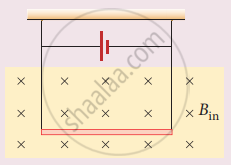Evaluation | Q IV. 3. | Page 192

A circular coil with a cross-sectional area 0.1 cm2 is kept in a uniform magnetic field of strength 0.2 T. If the current passing in the coil is 3 A and the plane of the loop is perpendicular to the direction of a magnetic field. Calculate

1. total torque on the coil
2. total force on the coil
3. average force on each electron in the coil due to the magnetic field. (The free electron density for the material of the wire is 1028 m-3).
Evaluation | Q IV. 4. | Page 193

A bar magnet is placed in a uniform magnetic field whose strength is 0.8 T. If the bar magnet is oriented at an angle 30° with the external field experiences a torque of 0.2 Nm. Calculate

1. the magnetic moment of the magnet
2. the work is done by the magnetic field in moving it from the most stable configuration to the most its configuration and also compute the work done by the applied magnetic field in this case.
Evaluation | Q IV. 5. | Page 193

A non – conducting sphere has a mass of 100 g and radius 20 cm. A flat compact coil of wire with turns 5 is wrapped tightly around it with each turns concentric with the sphere. This sphere is placed on an inclined plane such that plane of coil is parallel to the inclined plane. A uniform magnetic field of 0.5 T exists in the region in a vertically upward direction. Compute the current 1 required to rest the sphere in equilibrium.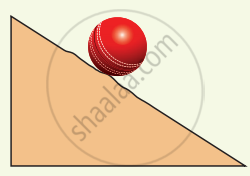Evaluation | Q IV. 6. | Page 193

Calculate the magnetic field at the center of a square loop which carries a current of 1.5 A, length of each loop is 50 cm.

## Chapter 3: Magnetism and magnetic effects of electric current

Evaluation## Tamil Nadu Board Samacheer Kalvi solutions for Class 12th Physics Volume 1 and 2 Answers Guide chapter 3 - Magnetism and magnetic effects of electric current

Tamil Nadu Board Samacheer Kalvi solutions for Class 12th Physics Volume 1 and 2 Answers Guide chapter 3 (Magnetism and magnetic effects of electric current) include all questions with solution and detail explanation. This will clear students doubts about any question and improve application skills while preparing for board exams. The detailed, step-by-step solutions will help you understand the concepts better and clear your confusions, if any. Shaalaa.com has the Tamil Nadu Board of Secondary Education Class 12th Physics Volume 1 and 2 Answers Guide solutions in a manner that help students grasp basic concepts better and faster.

Further, we at Shaalaa.com provide such solutions so that students can prepare for written exams. Tamil Nadu Board Samacheer Kalvi textbook solutions can be a core help for self-study and acts as a perfect self-help guidance for students.

Concepts covered in Class 12th Physics Volume 1 and 2 Answers Guide chapter 3 Magnetism and magnetic effects of electric current are Introduction to Magnetism, Coulomb’s Inverse Square Law of Magnetism, Torque Acting on a Bar Magnet in Uniform Magnetic Field, Magnetic Properties, Classification of Magnetic Materials, Hysteresis, Magnetic Effects of Current, Biot - Savart Law, Ampere’s Circuital Law, Lorentz Force, Torque on a Current Loop in Magnetic Field.

Using Tamil Nadu Board Samacheer Kalvi Class 12th solutions Magnetism and magnetic effects of electric current exercise by students are an easy way to prepare for the exams, as they involve solutions arranged chapter-wise also page wise. The questions involved in Tamil Nadu Board Samacheer Kalvi Solutions are important questions that can be asked in the final exam. Maximum students of Tamil Nadu Board of Secondary Education Class 12th prefer Tamil Nadu Board Samacheer Kalvi Textbook Solutions to score more in exam.

Get the free view of chapter 3 Magnetism and magnetic effects of electric current Class 12th extra questions for Class 12th Physics Volume 1 and 2 Answers Guide and can use Shaalaa.com to keep it handy for your exam preparation